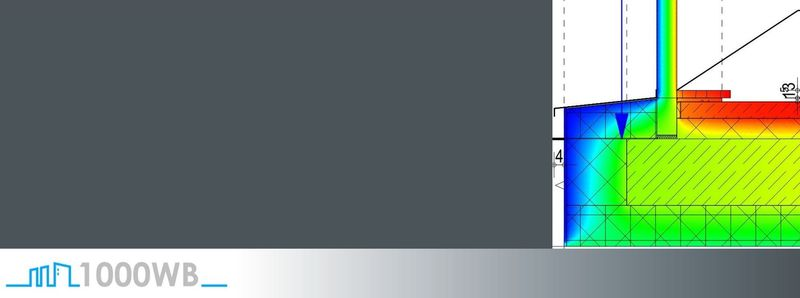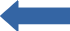# Reference lengths of the U-values – example window lintel

Posted on: May 8, 2020 Posted by: Jörg Gerl Comments: 0Here we have a thermal bridge that has a precisely defined thermal bridge coefficient for a given geometry Ψ.

This applies to the undisturbed area (the area which has no influence on the heat flow from adjacent components):
– U1: U-value, which is used in the heat requirement calculation as the U-value of the outer wall (in this example: 0.1331 W/m²K)
– U2: U-value that is used in the heat requirement calculation as the U-value of the window (in this example: 1.1005 W/m²K)

This geometry assumes that in the heat requirement calculation the undisturbed U-value, which is set for the window surface, extends to the upper edge of the window-wall junction.

However, if the window-wall junction is now included in the wall surface in the heat requirement calculation, the height of the window in this example is 6.5 cm smaller and the total surface of the window with the U-value in the heat requirement calculation, which is considerably worse than the wall, shrinks accordingly.

This reduces the calculated energy requirement of the entire building. If the reference lengths of the U-values used in the thermal bridge calculation were not adjusted, an error would result.

#### An example:

The dimensioning and reference lengths of the U-values correspond. It is not necessary to adjust the reference lengths of the U values.

Dimensioning and reference lengths of the U-values do not match. They differ by the dimension of the wall stop (6.5 cm).

This gives an incorrect result in the heat requirement calculation. The reference lengths of the U-values must be adjusted.

By adjusting the reference lengths of the U-values (here +6.5 cm for U1 and -6.5 cm for U2) an incorrect result of the heat requirement calculation is avoided.

Dimensioning and reference length of the U-values correspond again.

Let’s look at the matter arithmetically by inserting the values into the formula

ψ = L2D – (Σ Ui*lI*Fxi)

or here specifically:

ψ = L2D – (U1*l1*Fx1+ U2*l2*Fx2)

Case 1:
We have the following sizes in this example:

L2D = 0.6486
U1 = 0.1331 W/m²K
l1 = 1.165 m
Fx1 = 1
U2 = 1.1005 W/m²K
l2 = 0.500 m
Fx2 = 1

This results in Ψ = -0.0567 W/mK

Case 2:
If we now change the reference lengths of the U-values by the height of the window-wall junction (6.5 cm), it looks like this:

L2D = 0.6486
U1 = 0.1331 W/m²K
l1 = 1.165 m + 0.065 m = 1.230 m
Fx1 = 1
U2 = 1.1005 W/m²K
l2 = 0.500 m – 0.065 m = 0.435 m
Fx2 = 1

This results in Ψ = 0.0062 W/mK

This value is worse than the unchanged Ψ value and thus takes into account the fact that a smaller window area compared to the original case causes less energy loss.